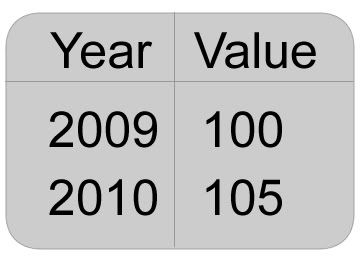# Use CPI to Calculate Inflation Rate

The Consumer Price Index (CPI ) which is a measure for inflation; it measures price increases and decreases consumer goods and services on a monthly basis. When the CPI goes up, it indicates an inflationary environment. Consumers have to pay more money for the same amount of goods and services.

Calculating the CPI Index is very simple. Let’s say in 2009 the price of goods is RM1.00. Now we have our consumer price index with a value of 100. It will become the base year for you to calculate the inflation rate. In 2010 the same goods now costs about RM1.05. So, our Consumer Price Index we have for the year 2010 is 105.

The Consumer Price Index will look something like:What you need to do is just subtract the most recent year’s CPI from the first year’s CPI. For example, if the CPI in the first year was 100 and the CPI from the most recent year was 105, then the result would be 5 (105 – 100 = 5).

After that, divide the result of by the CPI of the first year. (Example: 5 / 100 = 0.05). So, the inflation rate will be 5%.